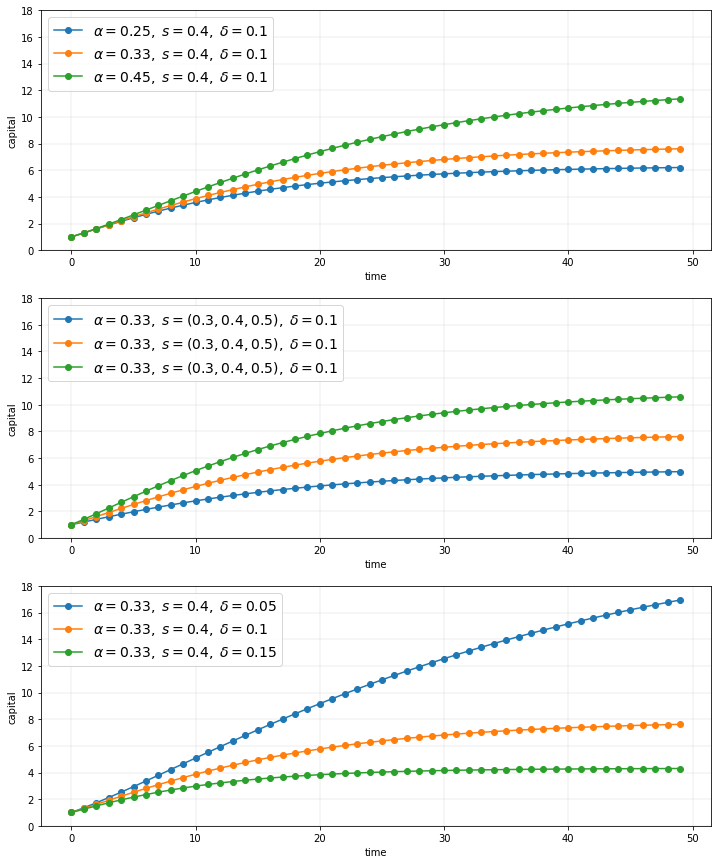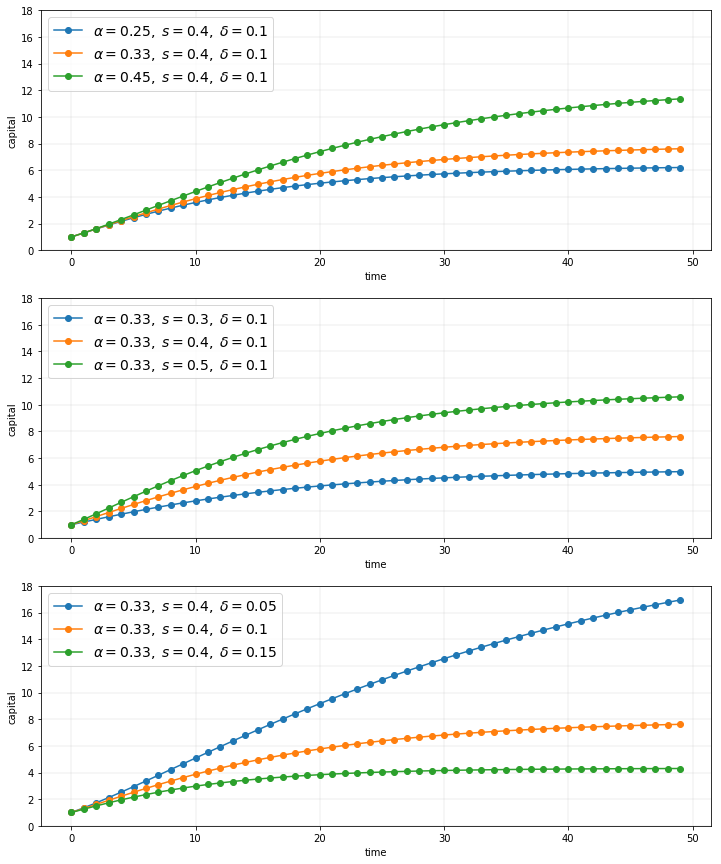We are working to support a site-wide PDF but it is not yet available. You can download PDFs for individual lectures through the download badge on each lecture page.

Code should execute sequentially if run in a Jupyter notebook

Writing Good Code¶

Overview¶

When computer programs are small, poorly written code is not overly costly

But more data, more sophisticated models, and more computer power are enabling us to take on more challenging problems that involve writing longer programs

For such programs, investment in good coding practices will pay high returns

The main payoffs are higher productivity and faster code

In this lecture, we review some elements of good coding practice

We also touch on modern developments in scientific computing — such as just in time compilation — and how they affect good program design

Let’s have a look at some poorly written code

The job of the code is to generate and plot time series of the simplified Solow model

$$k_{t+1} = s k_t^{\alpha} + (1 - \delta) k_t, \quad t = 0, 1, 2, \ldots \tag{1}$$

Here

• $k_t$ is capital at time $t$ and
• $s, \alpha, \delta$ are parameters (savings, a productivity parameter and depreciation)

For each parameterization, the code

1. sets $k_0 = 1$
2. iterates using (1) to produce a sequence $k_0, k_1, k_2 \ldots , k_T$
3. plots the sequence

The plots will be grouped into three subfigures

In each subfigure, two parameters are held fixed while another varies

In :
import numpy as np
import matplotlib.pyplot as plt
%matplotlib inline

# Allocate memory for time series
k = np.empty(50)

fig, axes = plt.subplots(3, 1, figsize=(12, 15))

# Trajectories with different α
δ = 0.1
s = 0.4
α = (0.25, 0.33, 0.45)

for j in range(3):
k = 1
for t in range(49):
k[t+1] = s * k[t]**α[j] + (1 - δ) * k[t]
axes.plot(k, 'o-', label=rf"$\alpha = {α[j]},\; s = {s},\; \delta={δ}$")

axes.grid(lw=0.2)
axes.set_ylim(0, 18)
axes.set_xlabel('time')
axes.set_ylabel('capital')
axes.legend(loc='upper left', frameon=True, fontsize=14)

# Trajectories with different s
δ = 0.1
α = 0.33
s = (0.3, 0.4, 0.5)

for j in range(3):
k = 1
for t in range(49):
k[t+1] = s[j] * k[t]**α + (1 - δ) * k[t]
axes.plot(k, 'o-', label=rf"$\alpha = {α},\; s = {s},\; \delta={δ}$")

axes.grid(lw=0.2)
axes.set_xlabel('time')
axes.set_ylabel('capital')
axes.set_ylim(0, 18)
axes.legend(loc='upper left', frameon=True, fontsize=14)

# Trajectories with different δ
δ = (0.05, 0.1, 0.15)
α = 0.33
s = 0.4

for j in range(3):
k = 1
for t in range(49):
k[t+1] = s * k[t]**α + (1 - δ[j]) * k[t]
axes.plot(k, 'o-', label=rf"$\alpha = {α},\; s = {s},\; \delta={δ[j]}$")

axes.set_ylim(0, 18)
axes.set_xlabel('time')
axes.set_ylabel('capital')
axes.grid(lw=0.2)
axes.legend(loc='upper left', frameon=True, fontsize=14)

plt.show()True, the code more or less follows PEP8

At the same time, it’s very poorly structured

Let’s talk about why that’s the case, and what we can do about it

Good Coding Practice¶

There are usually many different ways to write a program that accomplishes a given task

For small programs, like the one above, the way you write code doesn’t matter too much

But if you are ambitious and want to produce useful things, you’ll write medium to large programs too

In those settings, coding style matters a great deal

Fortunately, lots of smart people have thought about the best way to write code

Here are some basic precepts

Don’t Use Magic Numbers¶

If you look at the code above, you’ll see numbers like 50 and 49 and 3 scattered through the code

These kinds of numeric literals in the body of your code are sometimes called “magic numbers”

This is not a complement

While numeric literals are not all evil, the numbers shown in the program above should certainly be replaced by named constants

For example, the code above could declare the variable time_series_length = 50

Then in the loops, 49 should be replaced by time_series_length - 1

• the meaning is much clearer throughout
• to alter the time series length, you only need to change one value

Don’t Repeat Yourself¶

The other mortal sin in the code snippet above is repetition

Blocks of logic (such as the loop to generate time series) are repeated with only minor changes

This violates a fundamental tenet of programming: Don’t repeat yourself (DRY)

• Also called DIE (duplication is evil)

Yes, we realize that you can just cut and paste and change a few symbols

But as a programmer your aim should be to automate repetition, not do it yourself

More importantly, repeating the same logic in different places means that eventually one of them will likely be wrong

We’ll talk about how to avoid repetition below

Minimize Global Variables¶

Sure, global variables (i.e., names assigned to values outside of any function or class) are convenient

Rookie programmers typically use global variables with abandon — as we once did ourselves

But global variables are dangerous, especially in medium to large size programs, since

• they can affect what happens in any part of your program
• they can be changed by any function

This makes it much harder to be certain about what some small part of a given piece of code actually commands

Here’s a useful discussion on the topic

While the odd global in small scripts is no big deal, we recommend that you teach yourself to avoid them

(We’ll discuss how just below)

JIT Compilation¶

In fact, there’s now another good reason to avoid global variables

In scientific computing, we’re witnessing the rapid growth of just in time (JIT) compilation

JIT compilation can generate excellent performance for scripting languages like Python and Julia

But the task of the compiler used for JIT compilation becomes much harder when many global variables are present

(This is because data type instability hinders the generation of efficient machine code — we’ll learn more about such topics later on)

Use Functions or Classes¶

Fortunately, we can easily avoid the evils of global variables and WET code

• WET stands for “we love typing” and is the opposite of DRY

We can do this by making frequent use of functions or classes

In fact, functions and classes are designed specifically to help us avoid shaming ourselves by repeating code or excessive use of global variables

Which one, functions or classes?¶

Both can be useful, and in fact they work well with each other

(Personal preference is part of the story too)

What’s really important is that you use one or the other or both

Revisiting the Example¶

Here’s some code that reproduces the plot above with better coding style

It uses a function to avoid repetition

Note also that

• global variables are quarantined by collecting together at the end, not the start of the program
• magic numbers are avoided
• the loop at the end where the actual work is done is short and relatively simple
In :
from itertools import product

def plot_path(ax, αs, s_vals, δs, series_length=50):
"""
Add a time series plot to the axes ax for all given parameters.
"""
k = np.empty(series_length)

for (α, s, δ) in product(αs, s_vals, δs):
k = 1
for t in range(series_length-1):
k[t+1] = s * k[t]**α + (1 - δ) * k[t]
ax.plot(k, 'o-', label=rf"$\alpha = {α},\; s = {s},\; \delta = {δ}$")

ax.grid(lw=0.2)
ax.set_xlabel('time')
ax.set_ylabel('capital')
ax.set_ylim(0, 18)
ax.legend(loc='upper left', frameon=True, fontsize=14)

fig, axes = plt.subplots(3, 1, figsize=(12, 15))

# Parameters (αs, s_vals, δs)
set_one = ([0.25, 0.33, 0.45], [0.4], [0.1])
set_two = ([0.33], [0.3, 0.4, 0.5], [0.1])
set_three = ([0.33], [0.4], [0.05, 0.1, 0.15])

for (ax, params) in zip(axes, (set_one, set_two, set_three)):
αs, s_vals, δs = params
plot_path(ax, αs, s_vals, δs)

plt.show()Summary¶

Writing decent code isn’t hard

It’s also fun and intellectually satisfying

We recommend that you cultivate good habits and style even when you write relatively short programs

• Share page